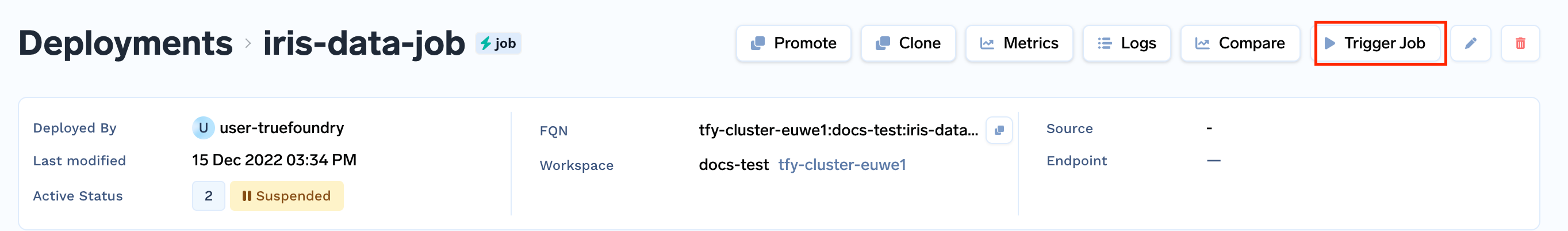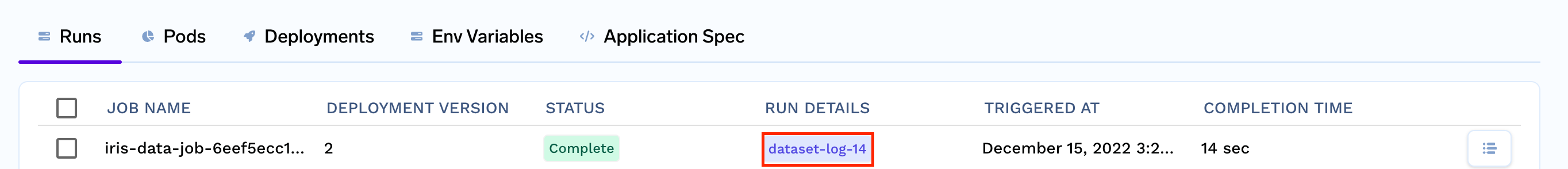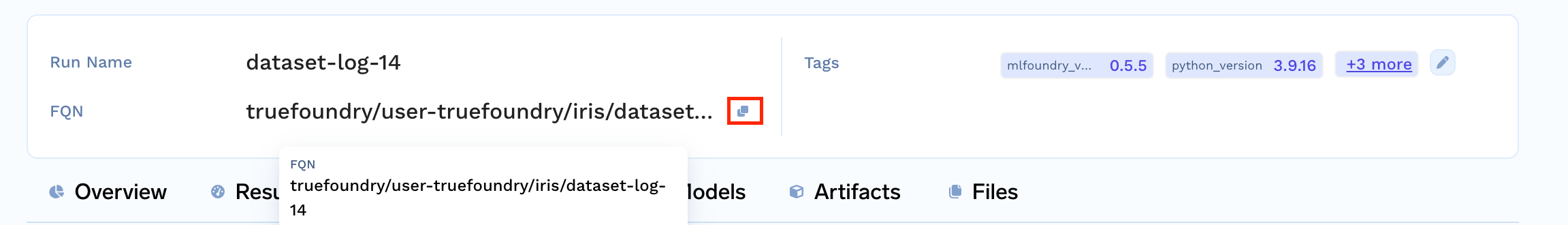# Log dataset and retrieve it back

👍

What you'll learn

• Log preprocessed dataset
• Retrieve the dataset back

This is a guide to log a preprocessed dataset via `mlfoundry` and then retrieve it back

## Project structure

To complete this guide, you are going to create the following files:

• `train.py` : contains our dataset logging code
• `deploy.py`: contains our deployment code
• `requirements.txt` : contains our dependencies

Your final file structure is going to look like this:

``````.
├── train.py
├── deploy.py
└── requirements.txt
``````

As you can see, all the following files are created in the same folder/directory

## Step 1: Implement the training code.

The first step is to create a script that logs preprocessed dataset via `mlfoundry`

Create the `train.py` and `requirements.txt` files in the same directory where the model is stored.

``````.
├── train.py
└── requirements.txt
``````

📘

Note

Currently `run.log_dataset()` only supports features that have primitive data type values. Specifically one of these :-

• "integer"
• "floating"
• "mixed-integer-float"
• "categorical"
• "boolean"
• "integer"
• "string"

If your feature has datatypes other than this, you might want to consider saving the dataset via `run.log_artifact()`. Follow through the guide, the last section covers how to use the log_artifacts method instead.

### `train.py`

``````import pickle
from sklearn.linear_model import LogisticRegression
from sklearn.preprocessing import StandardScaler, LabelEncoder
from sklearn.model_selection import train_test_split
from sklearn.metrics import accuracy_score, f1_score, confusion_matrix, ConfusionMatrixDisplay
import matplotlib.pyplot as plt

import mlfoundry as mlf
client = mlf.get_client()

X = X.rename(
columns={
"sepal length (cm)": "sepal_length",
"sepal width (cm)": "sepal_width",
"petal length (cm)": "petal_length",
"petal width (cm)": "petal_width",
}
)

X_train, X_test, y_train, y_test = train_test_split(
X, y, test_size=0.2, random_state=42, stratify=y
)

run = client.create_run(project_name="iris", run_name="dataset-log")

#Scaling the numerical features
scaler = StandardScaler()

cont_col = ["sepal_length", "sepal_width", "petal_length", "petal_width"]
scaler.fit(X_train[cont_col])
X_train[cont_col] = scaler.transform(X_train[cont_col])
X_test[cont_col] = scaler.transform(X_test[cont_col])

#Saving the scaler as a pickle artifact
pickle.dump(scaler, open('scaler.pkl', 'wb'))

run.log_artifact(local_path="scaler.pkl", artifact_path="my-artifact")

run.log_dataset(
dataset_name='train',
features=X_train,
actuals=y_train
)

run.log_dataset(
dataset_name='test',
features=X_test,
actuals=y_test
)
``````

Click on the Open Recipe below to understand the `train.py`:

### `requirements.txt`

``````scikit-learn
pickle-mixin
mlfoundry
``````

## Step 2: Deploying as a Job

You can deploy the job on Truefoundry programmatically via our Python SDK.

Create a `deploy.py`, after which our file structure will look like this:

File Structure

``````.
├── train.py
├── deploy.py
└── requirements.txt
``````

### `deploy.py`

🚧

In the code below, ensure to replace "YOUR_WORKSPACE_FQN" in the last line with your WORKSPACE_FQN

``````# Replace `<YOUR_WORKSPACE_FQN>` with the actual value.
# in the form of secrets
import logging
from servicefoundry import Build, Job, PythonBuild

logging.basicConfig(level=logging.INFO)

# First we define how to build our code into a Docker image
image = Build(
build_spec=PythonBuild(
command="python train.py",
requirements_path="requirements.txt",
)
)
job = Job(
name="iris-train-job",
image=image,
env={"TFY_API_KEY": "YOUR_TFY_API_KEY"}
)
job.deploy(workspace_fqn = "YOUR_WORKSPACE_FQN")
``````

Follow the recipe below to understand the deploy.py file :

To deploy using Python API use:

``````python deploy.py
``````

Run the above command from the same directory containing the `train.py` and `requirements.txt` files.

📘

.tfyignore files

If there are any files you don't want to be copied to the workspace, like a data file, or any redundant files. You can use .tfyignore files in that case.

## Step 3: Trigger the job

Once the job has been built successfully, click on the trigger job on the top right corner, and then click on trigger job button at bottom.This will trigger your job to run, then you can click on the Run's tab, and you will see your training job running.

Once it is finished, you can click on the run details columns `dataset-log`In the top you will see the Run FQN, copy it.## Step 4: Retrieve the dataset

If you have `mlfoundry` setup you can run the following script to retrieve your dataset.

``````import mlfoundry

client = mlfoundry.get_client()
run = client.get_run("<RUN_FQN>")
train_dataset = run.get_dataset(dataset_name="train")
test_dataset = run.get_dataset(dataset_name="test")

train_X = train_dataset.features
train_y = train_dataset.actuals
test_X = test_dataset.features
test_y = test_dataset.actuals
``````

# Logging dataset via Artifacts

If your dataset's features have complex datatypes, such as numpy arrays, `run.log_dataset()` will not work.
In cases like that you can save you dataset as an artifact via `run.log_artifact()`.

## Modifications

First, we will modify the `train.py` file.
We will store the features and labels in pickle files, and save the pickle files as artifact via `run.log_artifact()`

### `train.py`

``````import pickle
from sklearn.preprocessing import StandardScaler
from sklearn.model_selection import train_test_split

import mlfoundry as mlf
client = mlf.get_client()

X = X.rename(
columns={
"sepal length (cm)": "sepal_length",
"sepal width (cm)": "sepal_width",
"petal length (cm)": "petal_length",
"petal width (cm)": "petal_width",
}
)

X_train, X_test, y_train, y_test = train_test_split(
X, y, test_size=0.2, random_state=42, stratify=y
)

run = client.create_run(project_name="iris", run_name="dataset-log")

#Scaling the numerical features
scaler = StandardScaler()

cont_col = ["sepal_length", "sepal_width", "petal_length", "petal_width"]
scaler.fit(X_train[cont_col])
X_train[cont_col] = scaler.transform(X_train[cont_col])
X_test[cont_col] = scaler.transform(X_test[cont_col])

#Saving the dataset as pickle files
pickle.dump(X_train, open('train_features.pkl', 'wb'))
pickle.dump(X_test, open('test_features.pkl', 'wb'))
pickle.dump(y_train, open('train_labels.pkl', 'wb'))
pickle.dump(y_test, open('test_labels.pkl', 'wb'))

run.log_artifact(local_path="train_features.pkl", artifact_path="my-artifact")
run.log_artifact(local_path="test_features.pkl", artifact_path="my-artifact")
run.log_artifact(local_path="train_labels.pkl", artifact_path="my-artifact")
run.log_artifact(local_path="test_labels.pkl", artifact_path="my-artifact")
``````

Follow the recipe below to understand the train.py file :

## Retrieving the dataset

Now to retrieve the dataset back we can run the following script

``````import mlfoundry

client = mlfoundry.get_client()
run = client.get_run("<RUN_FQN>")